1800-1023-196

+91-120-4616500

# Miller Indices

## Miller Indices

Planes in crystals are described by a set of integers (h, k and l) known as Miller indices. Miller indices of a plane are the reciprocals of the fractional intercepts of that plane on the various crystallographic axes. For calculating Miller indices, a reference plane, known as parametral plane, is selected having intercepts a, b and c along x, y and z-axes, respectively. Then, the intercepts of the unknown plane are given with respect to a, b and c of the parametral plane.

Thus, the Miller indices are: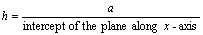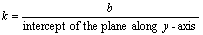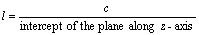The distance between the parallel planes in crystals are designated as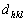. For different cubic lattices these interplanar spacing are given by the general formula,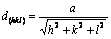Where a is the length of cube side while h, k and l are the Miller indices of the plane.

When a plane is parallel to an axis, its intercept with that axis is taken as infinite and the Miller will be zero.

Negative signs in the Miller indices is indicated by placing a bar on the intercept. All parallel planes have same Miller indices.

## NEET & AIIMS Exam Sample Papers

 AIIMS SAMPLE PAPERS View More NEET SAMPLE PAPERS View More